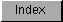```
FFP floating-point variables are defined within C by the float or FLOAT
directive. In assembly language they are simply defined by a DC.L/DS.L
statement. All FFP floating-point variables are defined as 32-bit entities
(longwords) with the following format:

_____________________________________________
|                                             |
| MMMMMMMM    MMMMMMMM    MMMMMMMM    EEEEEEE |
| 31          23          15          7       |
|_____________________________________________|

The mantissa is considered to be a binary fixed-point fraction; except for
0, it is always normalized (the mantissa is shifted over and the exponent
adjusted, so that the mantissa has a 1 bit in its highest position).
Thus, it represents a value of less than 1 but greater than or equal to
1/2.

The sign bit is reset (0) for a positive value and set (1) for a negative
value.

The exponent is the power of two needed to correctly position the mantissa
to reflect the number's true arithmetic value.  It is held in excess-64
notation, which means that the two's-complement values are adjusted upward
by 64, thus changing \$40 (-64) through \$3F (+63) to \$00 through \$7F.  This
facilitates comparisons among floating-point values.

The value of 0 is defined as all 32 bits being 0s.  The sign, exponent,
and mantissa are entirely cleared.  Thus, 0s are always treated as
positive.

The range allowed by this format is as follows:

DECIMAL:

9.22337177 * 10^18 > +VALUE > 5.42101070 * 10^-20
-9.22337177 * 10^18 < -VALUE < -2.71050535 * 10^-20